## Literals

The terms literal and constant value are synonymous and refer to a fixed data value. For example, 'JACK', 'BLUE ISLAND', and '101' are all character literals; 5001 is a numeric literal. Character literals are enclosed in single quotation marks, which enable Oracle to distinguish them from schema object names.

This section contains these topics:

Many SQL statements and functions require you to specify character and numeric literal values. You can also specify literals as part of expressions and conditions. You can specify character literals with the '`text`' notation, national character literals with the `N'text'` notation, and numeric literals with the `integer` or `number` notation, depending on the context of the literal. The syntactic forms of these notations appear in the sections that follow.

To specify a datetime or interval datatype as a literal, you must take into account any optional precisions included in the datatypes. Examples of specifying datetime and interval datatypes as literals are provided in the relevant sections of "Datatypes".

### Text Literals

Text specifies a text or character literal. You must use this notation to specify values whenever `'text'` or `char` appear in expressions, conditions, SQL functions, and SQL statements in other parts of this reference.

The syntax of text is as follows:

text::=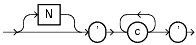Text description of text

where

• `N` specifies representation of the literal using the national character set. Text entered using this notation is translated into the national character set by Oracle when used.
• `c` is any member of the user's character set, except a single quotation mark (').
• ' ' are two single quotation marks that begin and end text literals. To represent one single quotation mark within a literal, enter two single quotation marks.

A text literal must be enclosed in single quotation marks. This reference uses the terms text literal and character literal interchangeably.

Text literals have properties of both the `CHAR` and `VARCHAR2` datatypes:

• Within expressions and conditions, Oracle treats text literals as though they have the datatype `CHAR` by comparing them using blank-padded comparison semantics.
• A text literal can have a maximum length of 4000 bytes.

Here are some valid text literals:

```'Hello'
'ORACLE.dbs'
'Jackie''s raincoat'
'09-MAR-98'
N'nchar literal'
```
 See Also: "About SQL Expressions" for the syntax description of `expr` "Blank-Padded Comparison Semantics"

### Integer Literals

You must use the integer notation to specify an integer whenever `integer` appears in expressions, conditions, SQL functions, and SQL statements described in other parts of this reference.

The syntax of `integer` is as follows:

integer::=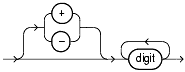Text description of integer

where `digit` is one of 0, 1, 2, 3, 4, 5, 6, 7, 8, 9.

An integer can store a maximum of 38 digits of precision.

Here are some valid integers:

```7
+255

```
 See Also: "About SQL Expressions" for the syntax description of `expr`

### Number Literals

You must use the number notation to specify values whenever `number` appears in expressions, conditions, SQL functions, and SQL statements in other parts of this reference.

The syntax of `number` is as follows:

number::=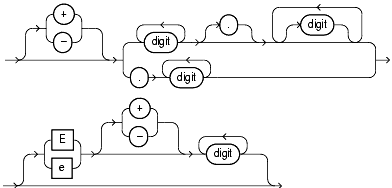Text description of number

where

• + or - indicates a positive or negative value. If you omit the sign, then a positive value is the default.
• `digit` is one of 0, 1, 2, 3, 4, 5, 6, 7, 8 or 9.
• e or E indicates that the number is specified in scientific notation. The digits after the E specify the exponent. The exponent can range from -130 to 125.

A `number` can store a maximum of 38 digits of precision.

If you have established a decimal character other than a period (.) with the initialization parameter `NLS_NUMERIC_CHARACTERS`, then you must specify numeric literals with `'text'` notation. In such cases, Oracle automatically converts the text literal to a numeric value.

For example, if the `NLS_NUMERIC_CHARACTERS` parameter specifies a decimal character of comma, specify the number 5.123 as follows:

```'5,123'
```

Here are some valid representations of `number`:

```25
+6.34
0.5
25e-03
-1
```
 See Also: "About SQL Expressions" for the syntax description of `expr`

### Interval Literals

An interval literal specifies a period of time. You can specify these differences in terms of years and months, or in terms of days, hours, minutes, and seconds. Oracle supports two types of interval literals, `YEAR` `TO` `MONTH` and `DAY` `TO` `SECOND`. Each type contains a leading field and may contain a trailing field. The leading field defines the basic unit of date or time being measured. The trailing field defines the smallest increment of the basic unit being considered. For example, a `YEAR` `TO` `MONTH` interval considers an interval of years to the nearest month. A `DAY` `TO` `MINUTE` interval considers an interval of days to the nearest minute.

If you have date data in numeric form, then you can use the `NUMTOYMINTERVAL` or `NUMTODSINTERVAL` conversion function to convert the numeric data into interval literals.

Interval literals are used primarily with analytic functions.

#### INTERVAL YEAR TO MONTH

Specify `YEAR` `TO` `MONTH` interval literals using the following syntax:

interval_year_to_month::=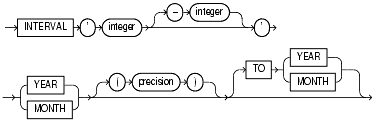Text description of interval_year_to_month

where

• `'integer [-integer]'` specifies integer values for the leading and optional trailing field of the literal. If the leading field is `YEAR` and the trailing field is `MONTH`, then the range of integer values for the month field is 0 to 11.
• `precision` is the maximum number of digits in the leading field. The valid range of the leading field precision is 0 to 9 and its default value is 2.
##### Restriction on the Leading Field

The leading field must be more significant than the trailing field. For example, `INTERVAL` '`0-1`' `MONTH` `TO` `YEAR` is not valid.

The following `INTERVAL` `YEAR` `TO` `MONTH` literal indicates an interval of 123 years, 2 months:

```INTERVAL '123-2' YEAR(3) TO MONTH

```

Examples of the other forms of the literal follow, including some abbreviated versions:

Form of Interval Literal Interpretation

`INTERVAL '123-2' YEAR(3) TO MONTH`

An interval of 123 years, 2 months. You must specify the leading field precision if it is greater than the default of 2 digits.

`INTERVAL '123' YEAR(3)`

An interval of 123 years 0 months.

`INTERVAL '300' MONTH(3)`

An interval of 300 months.

`INTERVAL '4' YEAR`

Maps to `INTERVAL '4-0' YEAR TO MONTH` and indicates 4 years.

`INTERVAL '50' MONTH`

Maps to `INTERVAL '4-2' YEAR TO MONTH` and indicates 50 months or 4 years 2 months.

`INTERVAL '123' YEAR`

Returns an error, because the default precision is 2, and '123' has 3 digits.

You can add or subtract one `INTERVAL` `YEAR` `TO` `MONTH` literal to or from another to yield another `INTERVAL` `YEAR` `TO` `MONTH` literal. For example:

```INTERVAL '5-3' YEAR TO MONTH + INTERVAL'20' MONTH =
INTERVAL '6-11' YEAR TO MONTH
```

#### INTERVAL DAY TO SECOND

Specify `DAY` `TO` `SECOND` interval literals using the following syntax:

interval_day_to_second::=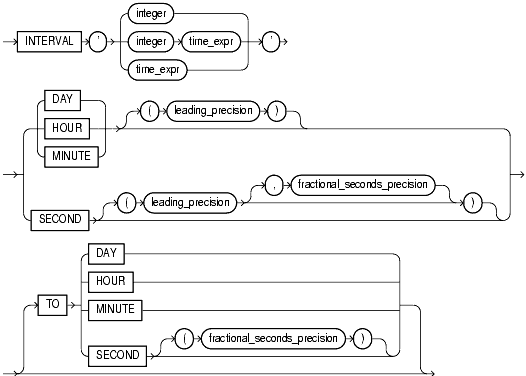Text description of interval_day_to_second

where

• `integer` specifies the number of days. If this value contains more digits than the number specified by the leading precision, then Oracle returns an error.
• `time_expr` specifies a time in the format HH[:MI[:SS[.n]]]or MI[:SS[.n]] or SS[.n], where `n` specifies the fractional part of a second. If `n` contains more digits than the number specified by `fractional_seconds_precision`, then `n` is rounded to the number of digits specified by the `fractional_seconds_precision` value. You can specify `time_expr` following an integer and a space only if the leading field is `DAY`.
• `leading_precision` is the number of digits in the leading field. Accepted values are 0 to 9. The default is 2.
• `fractional_seconds_precision` is the number of digits in the fractional part of the `SECOND` datetime field. Accepted values are 1 to 9. The default is 6.
##### Restriction on the Leading Field:

The leading field must be more significant than the trailing field. For example, `INTERVAL` `MINUTE` `TO` `DAY` is not valid. As a result of this restriction, if `SECOND` is the leading field, the interval literal cannot have any trailing field.

The valid range of values for the trailing field are as follows:

• `HOUR`: 0 to 23
• `MINUTE`: 0 to 59
• `SECOND`: 0 to 59.999999999

Examples of the various forms of `INTERVAL` `DAY` `TO` `SECOND` literals follow, including some abbreviated versions:

Form of Interval Literal Interpretation

`INTERVAL '4 5:12:10.222' DAY TO SECOND(3)`

4 days, 5 hours, 12 minutes, 10 seconds, and 222 thousandths of a second.

`INTERVAL '4 5:12' DAY TO MINUTE`

4 days, 5 hours and 12 minutes.

`INTERVAL '400 5' DAY(3) TO HOUR`

400 days 5 hours.

`INTERVAL '400' DAY(3)`

400 days.

`INTERVAL '11:12:10.2222222' HOUR TO SECOND(7)`

11 hours, 12 minutes, and 10.2222222 seconds.

`INTERVAL '11:20' HOUR TO MINUTE`

11 hours and 20 minutes.

`INTERVAL '10' HOUR`

10 hours.

`INTERVAL '10:22' MINUTE TO SECOND`

10 minutes 22 seconds.

`INTERVAL '10' MINUTE`

10 minutes.

`INTERVAL '4' DAY`

4 days.

`INTERVAL '25' HOUR`

25 hours.

`INTERVAL '40' MINUTE`

40 minutes.

`INTERVAL '120' HOUR(3)`

120 hours

`INTERVAL '30.12345' SECOND(2,4)`

30.1235 seconds. The fractional second '12345' is rounded to '1235' because the precision is 4.

You can add or subtract one `DAY` `TO` `SECOND` interval literal from another `DAY` `TO` `SECOND` literal. For example.

```INTERVAL'20' DAY - INTERVAL'240' HOUR = INTERVAL'10-0' DAY TO SECOND
```